# RapidPT

RapidPT is based on the work presented on this paper:

Accelerating Permutation Testing in Neuroimaging through Subspace Tracking: A new plugin for SnPM.

F. Gutierrez-Barragan, V.K. Ithapu, C. Hinrichs, C. Maumet, S.C. Johnson, T.E. Nichols, V. Singh.

Neuorimage, 2017.

1. References
2. Overview
3. Use Cases
4. Setup
5. Usage
6. Usage within SnPM
7. Code Organization
8. Related Work

## Overview

Multiple hypothesis testing is a problem in neuroimaging studies. Permutation testing is a nonparametric method for estimating a threshold that can identify what brain regions that display statistically significant differences or activity. The computational burden of this method, however, for low thresholds and large datasets can be prohibitive.

RapidPT is a MATLAB toolbox for fast, reliable, hardware independent, permutation testing. For ease of use we have integrated RapidPT into SnPM. Please refer to the Usage within SnPM section.

1. Fast: RapidPT has shown speedups ranging from 30-1000x faster than simple permutation testing implementations (left), and 2-40x faster than SnPM (right), a state of the art nonparametric testing toolbox in neuroimaging.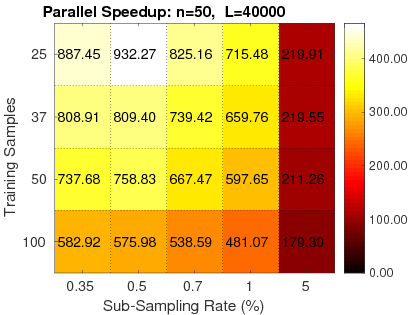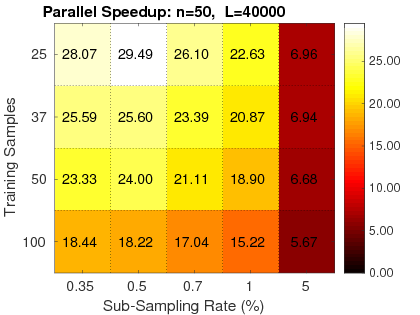2. Reliable: RapidPT has been validated against SnPM and a simple permutation testing implementation. Three validation measurements were used: the KL-Divergence between max null distributions, the corrected p-values, and the resampling risk. Hundreds of validation runs have been done with various neuroimaging datasets composed from 50 up to 400 subjects. For more information on the performance of RapidPT refer to the reference paper.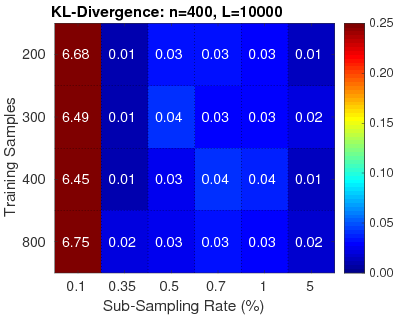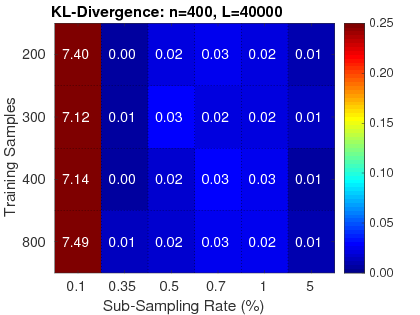3. Hardware Independent: It has been shown that with powerful enough hardware (highend GPUs or a cluster) and an efficient implementation, the permutation testing procedure can be spedup by orders of magnitude. These implementations rely on expensive hardware. RapidPT, however, takes advantage of the structure of the problem to speedup the algorithm, allowing it to be efficient even in regular workstations. Furthermore, the toolbox is able to leverage multi-core environments when available.

A thorough analysis of the scenarios were RapidPT performs best is done in the paper.

## Use cases

RapidPT can be used for the nonparametric statistical analysis of neuroimaging data. The permutation testing procedure modeled by RapidPT is a nonparametric combination of two-sample t-test. Two sample t-test are typically used to determine if two population means are equal. In neuroimaging this procedure could be used in scenarios such as placebo-control clinical trials or activation studies.

## Setup

Simply clone the repository and add the path of the repository inside your program to be able to call the functions.

``````addpath('PATH_WHERE_YOU_CLONED_THE_REPOSITORY');
%% YOUR MATLAB PROGRAM GOES HERE.
``````

If you don’t want to have the `addpath` line in every program you make, you can have it in your `startup.m` file for you MATLAB setup.

## Usage

RapidPT only offers a function that performs Permutation Testing. It has no GUI, pre or post processing modules. We have prepared a plugin for SnPM that allows the user to take advantage of SnPM’s GUI, pre and post processing capabilities. Please refer to the Usage Within SnPM section.

There are two ways to use the core of RapidPT, either by calling the wrapper function `TwoSampleRapidPT.m` or directly calling the core function `RapidPT.m`. `TwoSampleRapidPT` assigns some default inputs that have been extensively tested that produce an accurate recovery of the max null distribution and then calls `RapidPT`. On the other hand if you call `RapidPT` directly you will have to assign these parameters. Let’s first go through `Example_TwoSampleRapidPT.m`:

#### `Example_TwoSampleRapidPT.m`

1. First add the path to where you cloned/downloaded the `RapidPT` repository, and also load the data you will be working with. The data matrix needs to be an `NxV`, where `N` is the total number of subjects and V is the number of voxel statistics per subject.

`````` RapidPTLibraryPath = '.';
dataPath = 'PATH TO DATA';
``````
2. Set number of permutations and the number of subjects in either group 1 or 2 (it does not matter which one you specify).

`````` numPermutations = 5000;
nGroup1 = 25; % You should what is the size of one of your groups prior.
``````
3. Set `write`. If set to 0, outputs will only contain the constructed maximum null distribution. If set to 1, the outputs struct will contain the basis matrix, `U`, and coefficient matrix `W`. `U*W` recover the permutation matrix. For an in depth explanation see the references.

4. Call `TwoSampleRapidPT.m`. `outputs` is a struct containing `outputs.MaxT`,`outputs.U`, and `outputs.W`. `timings` is a struct containing timing information of different part of `RapidPT` as well as the total timing.

`````` [outputs, timings] = TwoSampleRapidPT(Data, numPermutations, nGroup1, write, RapidPTLibraryPath);
``````
5. Optionally save `outputs` and `timings`.

6. Get the t-threshold estimate from the recovered maximum null distribution.

`````` alpha_threshold = 1; % 1 percent
t_threshold = GetTThresh(outputs.MaxT, alpha_threshold);
``````
7. If you have the labels of the data available, you can calculate the two-sample t-test and see compare the result of each voxel to the t-threshold and see which voxels exhibit statistically significant activity.

#### `Example_RapidPT.m`

Take a look at the header comments of `RapidPT.m` and the comments in `Example_RapidPT.m` to see how to directly call `RapidPT.m`. It is recommended to use `TwoSampleRapidPT.m` in order to avoid hyperparameter tuning.

## Usage within SnPM

RapidPT is currently not available in the main SnPM repository. The plugin that we have developed is only available in our fork of the SnPM respository found here.

### Prerequistes

• SPM12 - In order to be able to use RapidPT within SPM/SnPM you will need to have SPM12 setup (obviously). For an overview of how to install SPM please refer to their wiki. If you have spm setup, running `spm` in the MATLAB command line should launch a GUI such as the one shown in the section snpm usage.

• NiFTI - You will also need the NiFTI toolset. Make sure the NiFTI toolset path is added before you run SnPM. `addpath('NiFTI toolset path')`.

• Git (recommended) - The setup below uses git to clone the repositories. Instead of cloning them you can also download the zip files from the links given throughout the setup instructions.

• RapidPT is only available for Two Sample t-test right now because it is the procedure that has been extensively validated and benchmarked. Regular SnPM should run if you try running SnPM with any other tests.

• For a thorough analysis of the ideas and the RapidPT algorithm please refer to the references

### SnPM + RapidPT Setup

Currently to use RapidPT within SnPM you will have to download the development version. To do this, go to wherever your SPM installation/folder is (mine is under my MATLAB folder) and do the following commands:

``````cd WHEREVER YOUR SPM DOWNLOAD IS
cd spm12/toolbox/
git clone https://github.com/felipegb94/SnPM-devel
cd SnPM-devel/
``````

### SnPM Usage

Now that you have setup RapidPT within SnPM, SnPM will work very similar to before. Launch SPM,

``````spm
``````

1. Go to SPM Menu window.
2. Click on Batch and go to the batch window that just opened.
SPM GUI SnPM Config + RPT Enable Option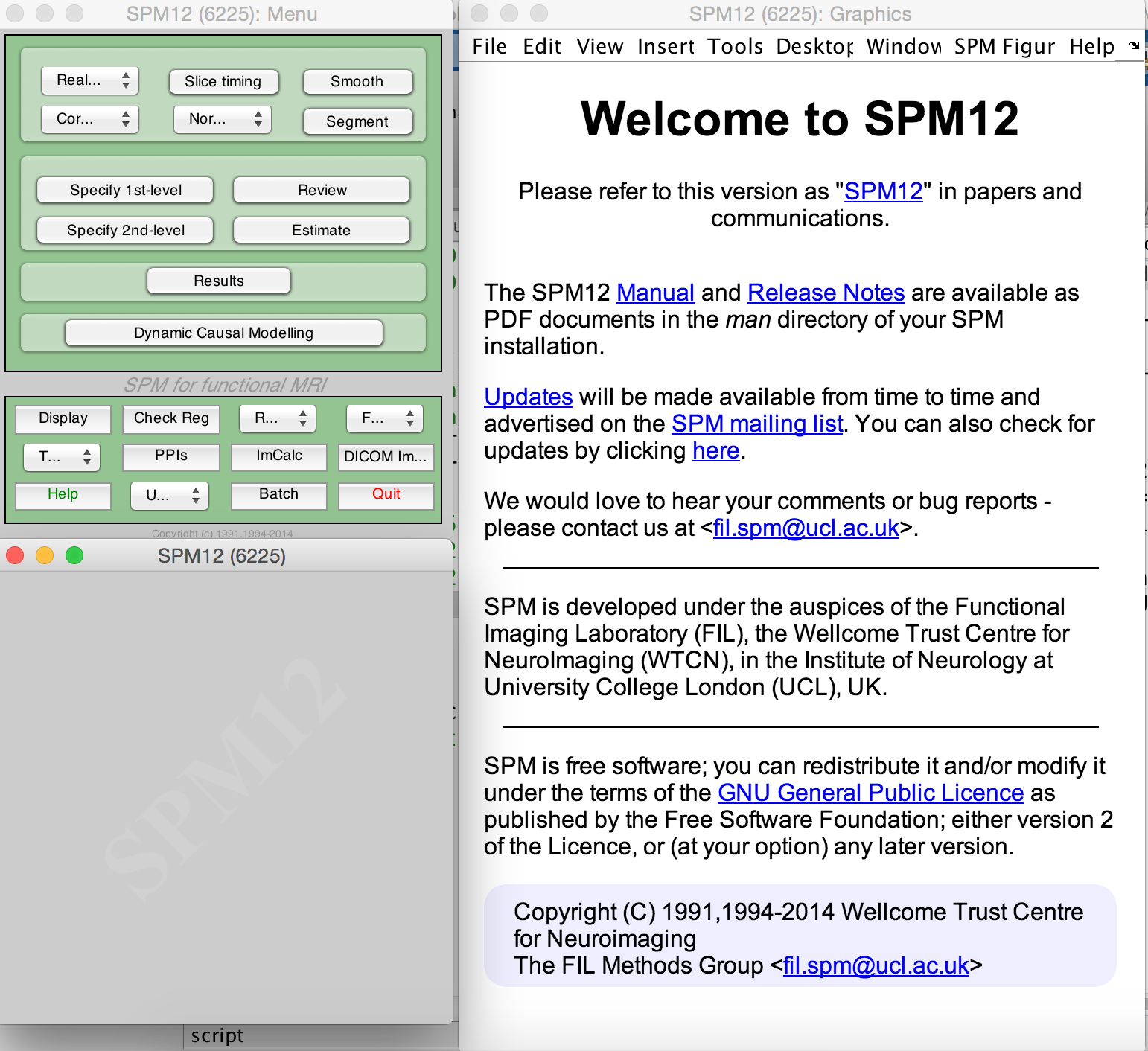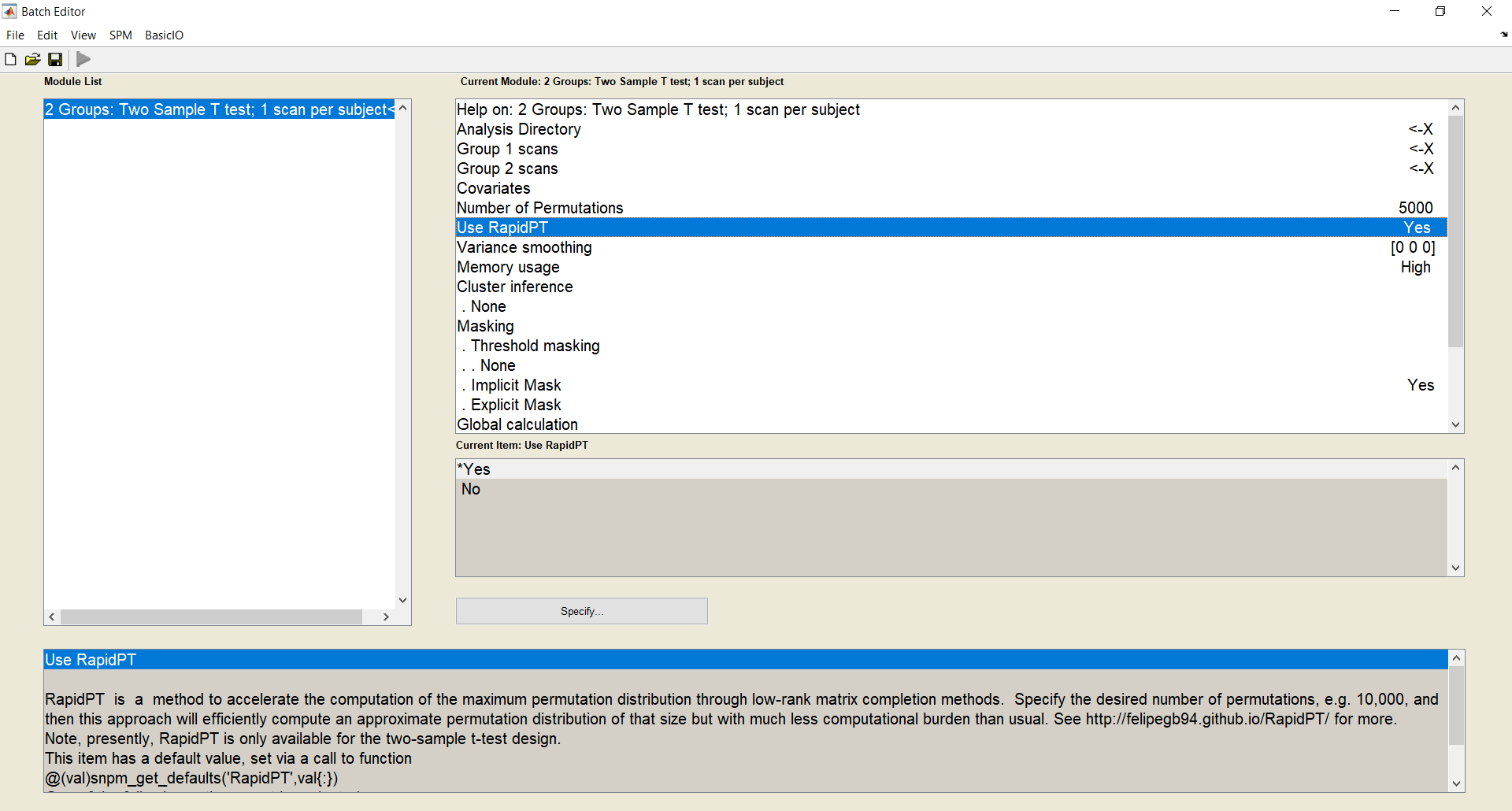1. On the navigation bar click on SPM, then `tools/SnPM/Specify/2 Groups Two Sample T test; 1 scan per subject`.
2. You can enable RapidPT by using the `Use RapidPT` option.
3. Here you will be able to specifiy a folder where you want your outputs to be (`Analysis Directory`), your input data (.nii images of group1 and group2), and also the number of permutations you want to do.
4. Click the green run button. This creates an SnPM config file in the path where you want your outputs to be. This step should take a few seconds only.
5. Go to SPM navigation bar again, then `Tools/SnPM/Compute`
6. Set the SnPM cfg file to the one you just made by clicking on the run button.
7. Click the green run button again, and now SnPM will run with RapidPT.
8. Once you are done, go to the directory that you selected as your `Analysis Directory` and look at the outputs.

### Post-processing and Outputs

Once you are done, inside your `analysis` directory you will find a folder called `outputs`. This folder contains the results. If you follow the results section of the (SnPM tutorial)[http://www2.warwick.ac.uk/fac/sci/statistics/staff/academic-research/nichols/software/snpm/man/ex] you can see an example of the post-processing capabilities of SnPM. The most important output files in the analysis directory will be:

• `SnPM.mat`: Refer to snpm_cp.m for a thorough explanations of the contents of this output file. But this file contains one of the objects of interest the variable `MaxT`, which is an nPerm x 2 matrix of [max;min] t-statistic i.e it contains the Max null distribution.
• `SnPMt.mat`: This is the resulting test statistic calculated using the original labels of the data.
• `XYZ.mat`: This matrix has the x, y, z coordinates associated to each voxel.
• `params.mat`: This structure contains the following parameters of the permutation testing run: nPerm (number of permutations), N (number of subjects), V (number of voxels after preprocessing), xdim, ydim, zdim.
• `timings.mat`: Contains some timing from rapidpt.
• `SnPMucp.mat`: Contains a 1 x NumVoxels matrix of the nonparametric P values of the statistic of interest supplied for all voxels at locations XYZ.

The following plot is a histogram of the Maxnull distribution in MaxT and a t-threshold associated to an alpha=0.05.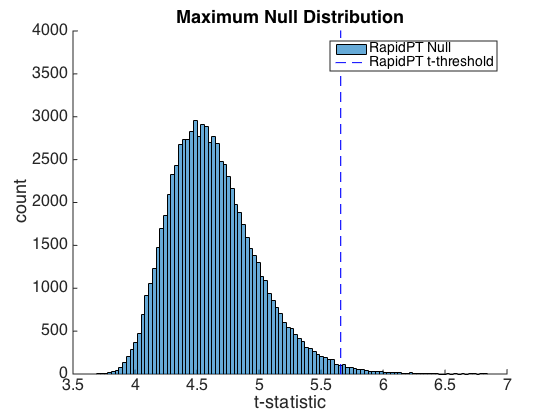## Code Organization

### RapidPT

#### `RapidPT.m`

This is the core of RapidPT. This is where the main algorithm and math ideas described in the NIPS paper happen.

#### `TwoSampleRapidPT.m`

This is a wrapper of the core. This function will assign most of the hyperparameters that can be given to `RapidPT.m` for you. The hyperparameters chosen have been extensively tested, and some of them are derived from the data dimensions and number of permutations chosen.

#### `Example_TwoSampleRapidPT.m`

This is an example script that uses `TwoSampleRapidPT.m` wrapper program.

#### `Example_RapidPT.m`

This is an example script that directly uses `RapidPT.m`. You will note that a lot more hyperparameters need to be passed to `RapidPT.m` compared to `TwoSampleRapidPT.m`.

#### `TwoSampleGetLabelsMatrices.m`

This is a function that given: `numPermutations` (Number of Permutations to be done), `N` (total number of subjects), `nGroup1` (The number of subjects in group1), returns the labels for each subject in each group that will be used at each permutation.

### include/

The `include/` folder contains the library grasta. This library contains the online matrix completion method we use to accelerate permutation testing. For more information about grasta you can refer to the project website.

### outputs/ & timings/

These are the default directories used to output the resulting max-null distribution, and timings of different sections of the algorithm. If the flag `inputs.write` is set to `1` the low-rank basis, `U`, and the coefficient matrix, `W`, that can recover the permutation matrix will also be saved.

### util/

This directory contains various utility functions used by RapidPT for input validation and post-processing. Separating these functions from the main code makes `TwoSampleRapidPT.m` more concise.

The main theorem motivating this work was presented in this conference paper:

C. Hinrichs, V. K. Ithapu, Q. Sun, S. C. Johnson, V. Singh.

Speeding up Permutation Testing in Neuroimaging

Advances in Neural Information Processing Systems (NIPS), 2013